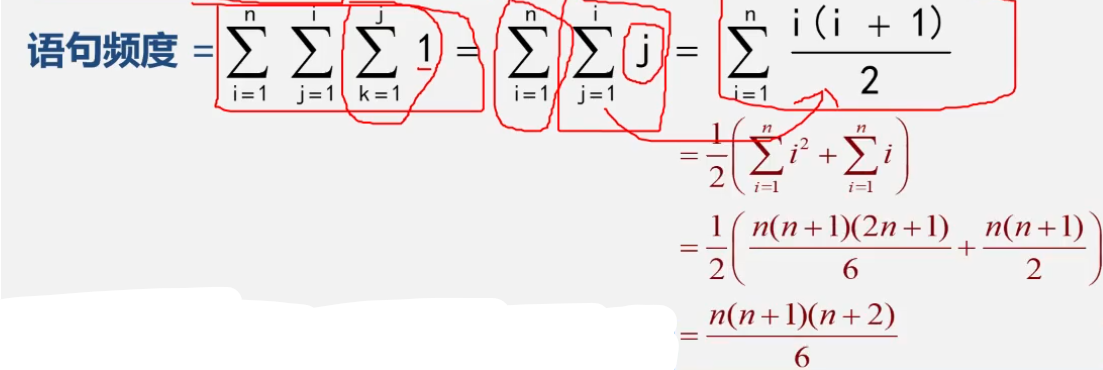• 记作T（n）=Of(n),称Of(n)为算法的渐进时间复杂度，简称时间复杂度。 分析算法中次数最多的语句就可以分析渐进时间复杂度。 4定理 取最高次数方为O（n） x=0;y=0 for (int i=1;i 时间复杂度是由嵌套最深层语句的频度...
1.算法定义：对特定问题求解方法和步骤的一种描述，它是指令的有限序列。其中每个指令表示一个或多个操作。
算法描述：自然语言 流程图 伪代码 程序代码
2.算法效率（好的算法具备正确性，健壮性和可读性）
时间效率：指的是算法所耗费的时间
空间效率：指的是算法执行过程中所耗费的存储空间
时间效率与空间效率有时候是矛盾的。
3.算法时间效率的度量：事后统计（将算法实现，测算时间）和事前分析（对算法耗费资源的一种估算）
算法运行时间=一个简单操作时间*简单操作次数
（每条语句频度 * 该语句执行一次所需的时间）
例如 （将一次时间看为单位时间）：
for (i=1;i<=n;i++)//n+1次
for(j=1;j<=n;j++){ //n(n+1)次
c[i][j]=0;//n*n次
for (k=0;k<n;k++)//n*n*(n+1)次
c[i][j]=c[i][j]+a[i][k]*b[k][i];//n*n*n次
}

若有某个辅助函数f(n),使得当n趋近于无穷大时，T(n)/f(n)的极限值为不等于零的常数，则称f（n）是T(n)的同数量级函数。记作T（n）=Of(n),称Of(n)为算法的渐进时间复杂度，简称时间复杂度。
分析算法中次数最多的语句就可以分析渐进时间复杂度。
4定理
取最高次数方为O（n）
x=0;y=0
for (int i=1;i<n;i++)//n+1次
x++;//n次
for(int j=1;j<n;j++){ //n+1次
for (int k=0;k<n;k++)//n*(n+1)次
y++;//n*n
}
T(n)=O(n平方)

时间复杂度是由嵌套最深层语句的频度决定。
for (i=1;i<=n;i++)//n+1次
for(j=1;j<=n;j++){ //n(n+1)次
c[i][j]=0;//n*n次
for (k=0;k<n;k++)//n*n*(n+1)次
c[i][j]=c[i][j]+a[i][k]*b[k][i];//n*n*n次
}
T(n)=O(n3次方)5渐进空间复杂度
算法要占据的空间：算法本身要占据的空间，输入，输出，指令，常数，变量等以及与算法所需要的辅助空间。
例如：
将一维数组中a中的n个数逆序放到原数组中
6.抽象数据类型=数据的逻辑结构+抽象运算（运算的功能描述）


展开全文算法
• 为什么算法渐进复杂度中对数的底数总为2 作者 张洋 | 发布于 2013-01-29 算法 时间复杂度 在分析各种算法时，经常看到O(log2n) 或O(nlog2n)这样的渐进复杂度。不知有没有同学困惑过，为什么算法的...
为什么算法渐进复杂度中对数的底数总为2

作者 张洋 | 发布于 2013-01-29
算法

时间复杂度

在分析各种算法时，经常看到O(log2n)

或O(nlog2n)这样的渐进复杂度。不知有没有同学困惑过，为什么算法的渐进复杂度中的对数都是以2为底？为什么没有见过O(nlog3n)
这样的渐进复杂度？本文解释这个问题。
三分式归并排序的时间复杂度
先看一个小例子。
大多数人应该对归并排序（merge sort）很熟悉，它的渐进复杂度为O(nlog2n)
。那么如果我们将归并排序改为均分成三份而不是两份，其算法时间复杂度是否有变化呢？
递归分析
下面通过递归分析对三分式归并排序的时间复杂度进行分析。因为不管是三分还是二分，对于总共n个数据来说，一遍合并的复杂度为O(n)
，所以三分式归并排序的递归式为：
T(n)=3T(n/3)+O(n)
如果把这个递归式的递归树画出来，很容易得到T(n)=O(nlog3n)
。如下图所示：对数的陷阱
那么这是否意味着三分式归并排序在时间复杂度上要优于二分式的归并排序呢？因为直觉上nlog3n
比nlog2n
要优一些。
实际上三分式归并排序的时间复杂度确实是T(n)=O(nlog3n)
，而且同时也是T(n)=O(nlog2n)
。
这看起来似乎是矛盾的，nlog3n
和nlog2n当然在绝大多数情况下是不相等的，但是在渐进复杂度情况下就不同了，因为渐进复杂度是忽略常系数的，但是似乎也看不出来nlog3n和nlog2n
是差一个常系数。关键就在于我们应该在中学学过的一个东西：对数换底公式。
logab=logcblogca
其中a和c均大于0且不等于1。
根据换底公式可以得出：
log3n=log2nlog23
所以nlog3n
比nlog2n只差一个常系数1log23
。因此，从渐进时间复杂度看，三分式归并并不比二分式归并更优，当然还是有个常系数的差别的。
更一般的：
logan=log2nlog2a
因此对于大于1的a来说，都与O(log2n)
差一个常系数而已，因此为了简便，一般都用O(log2n)表示对数的渐进复杂度，这就解决了本文初始的疑问。当然，以任何大于1的a为底数都是没有问题的。

展开全文• 计算算法渐进复杂度 找到语句频度最大的基本语句 写出该语句的 f(n) (n为执行次数,n可以是表达式) 忽略低次幂项和最高次项的系数 去数量级用"O"表示例1) f(m*n)--->O(m*n)例2) for(i=1;i<=n;i++) for(j=1...
计算算法渐进复杂度

找到语句频度最大的基本语句
写出该语句的   f(n)  (n为执行次数,n可以是表达式)
忽略低次幂项和最高次项的系数
去数量级用"O"表示例1)     f(m*n)--->O(m*n)例2)

for(i=1;i<=n;i++)
for(j=1;j<=i;j++)
for(k=1;k<=j;k++)
x+=1;j个1的和==j

高斯求和=(首相+末项)项数/2==i(i+1)/2

套公式化简

例3)

i=1;

while(i<=n)

i=i*2;

循环x次 : i=2^x

i<=n   2^x<=n   x<=log2(n)

f(n) = log2(n)

T(n)=O(log n)


展开全文• 没错，那就是关于复杂度的分析，真的是太难了，要对复杂度有一个深刻的理解，我觉得首先要深刻理解计算机系统。即便如此，我也还是得写一点简单的总结, 万事都是要开头，你才会继续去做的！ 常数O(1) 这里有一...
最近杨振宁和姚期智的回到中国国籍的事情非常热闹，作为一个在中国想做coding相关工作的人，你就不应该不知道清华姚班这个神奇的存在。老子这辈子是没有一丝机会达到那个高度了，但是我要努力使我儿子有机会够到那个高度，哈哈哈，开玩笑。但是，这和这个章节的题目有什么关系呢？那我因为我早上在知乎上看了一下Aady Yao的研究成果：

早在博士就读期间，姚期智提出了随机化算法复杂度的论证，而如今已经成为研究者无人不知的重要工具。
在 1977 年的论文中，姚期智提出了Yao's min-max principle，这一原理成为了推理随即算法与复杂度的基本技术，也已经应用于属性测试与学习理论等领域。

没错，那就是关于复杂度的分析，真的是太难了，要对复杂度有一个深刻的理解，我觉得首先要深刻理解计算机系统。即便如此，我也还是得写一点简单的总结, 万事都是要开头，你才会继续去做的！

常数O(1)
这里有一个简单的问题，我们要对三个数进行排序，并输出最大元素。不妨取前三个元素x=S, y=S, z=S, 这一步只需要执行三次(从特定单元提取元素)，耗费O(3)时间。接下来，为确定这三个元素的的大小顺序，最多需要三次排序，也是O(3)时间，最后输出最大的元素需要O(1)时间。

T(n) =O(3)+O(3)+O(1)=O(7)=O(1)

运算时间可表示和度量为T(n)=O(1)的这一类算法，统称为”常数时间复杂度算法”，也称为就地算法。

对数O(logn<!--//--><![CDATA[//><!--
logn
//--><!]]>)
考察如下问题：对于任意非负整数，统计其二进制展开中位数1的总数。

int countOnes(unsigned int n){
int ones=0；
while(n>0){
ones+=(1&n);
n>>1;
}
}

根据右移运算的性质，没右移一位，n至少缩减一半，也就是说，至多经过1+log2n<!--//--><![CDATA[//><!--
log_2n
//--><!]]>次循环，n必然缩减至0，从而算法终止。实际上从另一个角度上讲，1+log2n<!--//--><![CDATA[//><!--
log_2n
//--><!]]>恰好为n二进制展开的总位数，每次循环都将其右移一位，总的循环数也应是1+log2n<!--//--><![CDATA[//><!--
log_2n
//--><!]]>。
所以countOnes的算法执行时间主要由循环的次数决定：
O(1+log2n<!--//--><![CDATA[//><!--
log_2n
//--><!]]>)=O(log2n<!--//--><![CDATA[//><!--
log_2n
//--><!]]>)
在对数时间复杂度里面，log2n<!--//--><![CDATA[//><!--
log_2n
//--><!]]>实际上与底数无关，底数2可以换成其他任何数。

线性O(n)
关于线性时间复杂度，我们可以改进一下countOne算法：

int countOnes(unsigned int n){
int ones=0；
while(n>0){
count++
n&=n-1；
}
}

这个算法巧妙地通过位运算技巧，自低向高逐个地将位数1转置为0。
以上计算过程中，仅涉及整数的减法和位与运算各一次。若不考虑机器的位长限制，各只需要O(1)时间因此，这个新算法的运行时间，应线性正比于n的二进制展开中位数1的实际数目。

指数O(2n<!--//--><![CDATA[//><!--
2^{n}
//--><!]]>)


--int64 power2BF_I(int n){
__int64 pow=1;
while(n-- >0){
pow<<=1;
return pow;
}

这里的power2BF_I算法是由n轮迭代组成的，各需做一次累乘和一次递减，均属于基本操作，故整个算法时间共需O(n)时间。但是若以输入指数n的二进制位数r=1+log2n<!--//--><![CDATA[//><!--
log_2n
//--><!]]>作为输入规模，则运行时间为O(2n<!--//--><![CDATA[//><!--
2^{n}
//--><!]]>)。
展开全文• 2.分为常数阶时间复杂度即O(1);多项式阶即O(n^5);指数阶和对数阶 3.运算规则： O(f)+O(g)=O(max(f,g)) O(f)+O(g)=O(f+g) O(f)O(g)=O(fg) O(f)=f 4.求解递归式的三种方法： 代入法即先猜测，再用数学归纳法验证 用...算法
• BSP算法渐进复杂度的推导 直接利用主定理对形如T(n)=a*T(n/b)+f(n)的递归式设f(1)=O(1)并设物体均匀分布取a=7/6（只是估计，想象leaf为一立方体）b=2f(n)=1K=1则有a*f(n/b)>=K*f(n)所以T(n)=O(n^...
• 渐进紧确 界， big-Omega 表示的是下界。 2、 (1)Θ（西塔）：紧确界。  相当于"=" (2)O （大欧）：上界。 相当于" (3)o（小欧）：非紧的上界。 相当于" (4)Ω（大欧米伽）：下界。 ...算法
• 对于某些问题，一些算法更适合于用小规模的输入，而另一些则相反。...这种着眼长远，更为关注时间复杂度的总体变化趋势和增长速度的策略和方法，即所谓的渐进分析（asymptomatic analysis）。大 OO
•  可以使用大O记号表示一个算法的时间复杂度。下列表示不正确的是  D 。   百思不得其解之下，无奈百度，最后终于在 http://tieba.baidu.com/p/749296181 （五楼，六楼）找到答案。 ...数据结构 算法
• ## 渐进时间复杂度

千次阅读 2018-07-10 18:31:48
概念 渐进时间复杂度(时间复杂度)是算法效率的度量。在一般情况下，算法基本操作重复执行的次数用T(n)表示。同时用一个辅助函数f(n)，T(n)/f(n)!=0，那么f(n)是T(n)的同数量级函数，T(n)=O(f(n))，这就称为渐进时间...
• 这里写自定义目录标题渐进时间复杂度（O,o）依赖数学定义[^1]关于定义中的c 渐进时间复杂度（O,o） 这里通过从无穷大的比较来理解，渐进时间复杂度。 要求具有高等数学基础 依赖 可以从无穷大比较分析的原因： ...算法
• 渐进的时间复杂度  计算程序步数的目的是想比较两个或多个完成相同功能的程序的时间复杂度，并估计当问题规模变化时，程序的运行时间如何随之变化。  要确定一个程序的准确的程序步数是非常...数据结构
• 分析算法时间复杂度的基本方法 1.找出语句频度最大的那条语句作为基本语句； 2.计算基本语句的频度，得到问题规模n的某一个函数； 3.取其数量级用O表示 忽略所有低次幂项和最高次幂的系数，这样可以简化算法分析，...
• 前言：此篇章慢慢的引入如何比较两个算法时间复杂度的比较方法。 想学好算法 必须要理解好时间复杂度这个基本概念。...T(n) = O（f(n)），O为算法的渐进时间的复杂度，简称为时间复杂度。 趣味看四个场算法 数据结构 c语言
• 渐进复杂度分析概念目的大O表示法复杂度分析方法常用复杂度级别复杂度分析的4个概念 概念 复杂度描述的是算法执行时间（或占用空间）与数据规模的增长关系，描述了当数据量趋近于无穷大的时候，算法的执行时间或...算法 学习笔记
• ...   数据结构和算法本身解决的是“快”和“省”的问题，即如何让代码运行地更快，如何让代码更省存储空间。所以，执行效率是算法一个非常重要的...时间复杂度，全称：渐进时间复杂度 空间复杂度，全称：渐进空间...数据结构 算法
• statement 1~statement 5的执行次数分别为n+1,n(n+1),n^2,(n+1)n^2,n^3,算法时间复杂度： T(n) = 2*n^3+3*n^2+2*n+1 当问题的规模n趋向无穷大时等于2 lim[T(n)/n^3] = 2 所以，T(n)= O(n^3)C++
• i = Math.pow(i, c)) { // do O(1) } 上述代码中，循环变量 i 的初始值为 2，i 的值变化如下及循环的时间复杂度计算如下： 我们最终忽略低阶及常数阶之后得到的渐进时间复杂度为 O(LogLogn) O(nLogn): 下面我们来看...
• 渐进符号是分析算法时间复杂度的常用记号，对于某个规模为n的问题，当n足够大时，就可以忽略掉复杂度表达式中的低阶项和最高次项的系数，由此引出“渐进复杂度”，并且用渐进符号来对“渐进复杂度”进行表达。...
• c 表示常数 上述代码中，循环变量 i 的初始值为 2，i 的值变化如下及循环的时间复杂度计算如下： 我们最终忽略低阶及常数阶之后得到的渐进时间复杂度为 O(LogLogn) O(nLogn): 下面我们来看一个渐进时间复杂度为 O......